### Home > CALC > Chapter 10 > Lesson 10.3.1 > Problem10-118

10-118.
1. Examine the integrals below. Consider the multiple tools available for integrating and use the best strategy. After evaluating each integral, write a short description of your method. Homework Help ✎

1.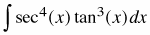2.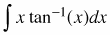3.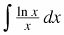4.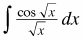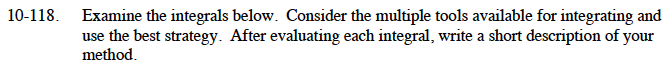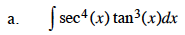Rewrite the integrand in terms of sine and cosine and use the Pythagorean Identity.

$=\int\frac{(1-\cos^2(x))\sin(x)}{\cos^7(x)}dx$

Let u = cos(x).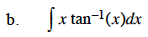Use integration by parts: Let f = tan–1(x) and dg = x dx

$\int x\tan^{-1}(x)dx=\frac{1}{2}x^2\tan^{-1}(x)-\frac{1}{2}\int \frac{x^2}{1+x^2}dx$

Use polynomial division:

$=\frac{1}{2}x^2\tan^{-1}(x)-\frac{1}{2}\int \Big(1-\frac{1}{1+x^2}\Big)dx$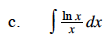Use substitution: Let u = ln(x)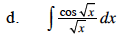Use substitution:

$\text{Let }u=\sqrt{x}$

$df=\frac{1}{1+x^2}\text{, }g=\frac{1}{2}x^2$